# Lami's Theorem

Lami’s theorem relates the magnitudes of coplanar, concurrent and non-collinear forces that maintain an object in static equilibrium. The theorem is very useful in analyzing most of the mechanical as well as structural systems.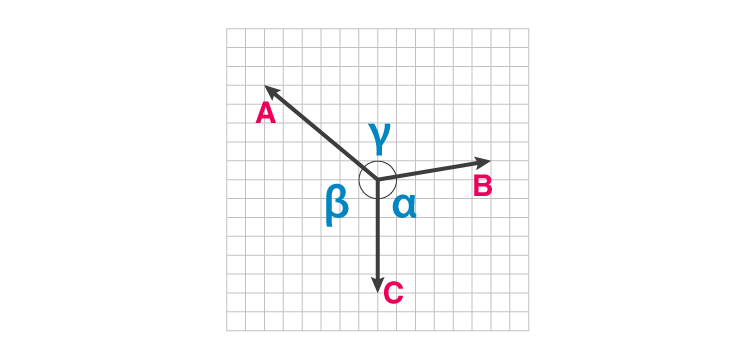## Lami’s Theorem Statement

Lami’s Theorem states, “When three forces acting at a point are in equilibrium, then each force is proportional to the sine of the angle between the other two forces”. Referring to the above diagram, consider three forces A, B, C acting on a particle or rigid body making angles α, β and γ with each other.

In the mathematical or equation form, it is expressed as,

$\frac{A}{sin\alpha} = \frac{B}{sin\beta} = \frac{C}{sin\gamma}$

## Lami’s Theorem Derivation

Now, let’s see how the theorem’s equation is derived.

Let FA, FB, and FC be the forces acting at a point. As per the statement of the theorem, we take the sum of all forces acting at a given point which will be zero.

i.e. FA + FB + FC = 0

The angles made by force vectors when a triangle is drawn are,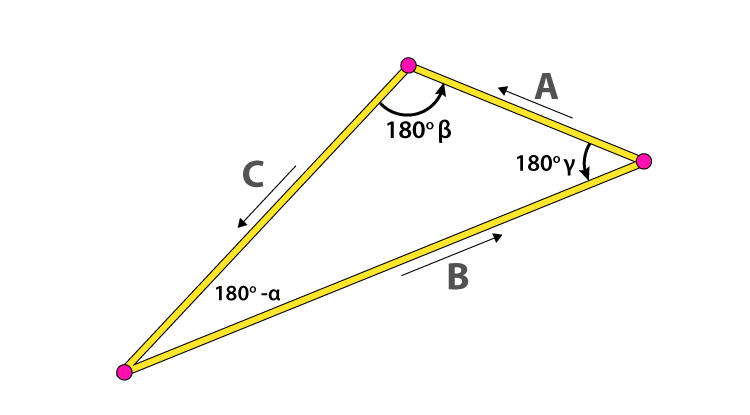We write angles in terms of complementary angles and use triangle law of vector addition. Then, by applying the sine rule we get,

$\frac{A}{sin(180-\alpha)} = \frac{B}{sin(180-\beta)} = \frac{C}{sin(180-\gamma)}$

So, we have,

$\frac{A}{sin\alpha} = \frac{B}{sin\beta} = \frac{C}{sin\gamma}$

Hence, it is clearly seen that by applying sine rule to complementary angles we arrive at the required result for Lami’s theorem.

Now, we will see how Lami’s theorem is useful to determine the magnitude of unknown forces for the given system.

## Lami’s Theorem Problems and Solved Examples

Example 1: Consider an advertisement board hangs with the help of two strings making an equal angle with the ceiling. Calculate the tension in both the strings in this case.Solution: The free body diagram of the same helps us to resolve the forces first. After resolving the forces we will apply the required theorem to get the value of tension in both the strings. Here, the weight of the signboard is in a downward direction, and the other force is the tension generated by the signboard in both the strings. In this case, the tension T in both the strings will be the same as the angle made by them with the signboard is equal.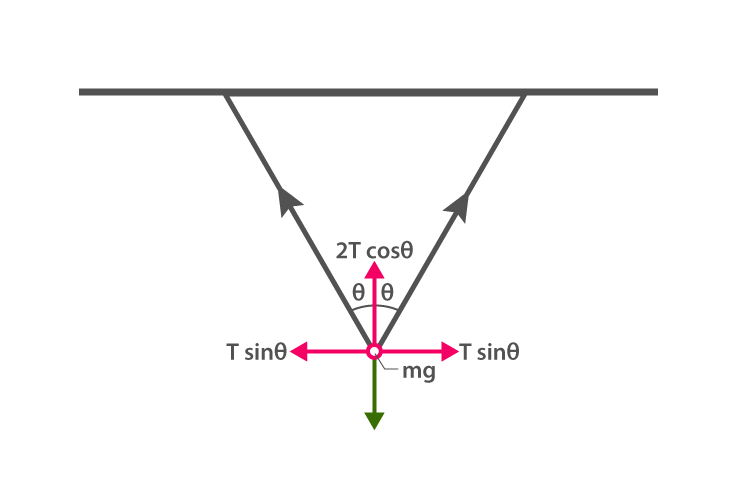Above figure represents the free body diagram of the signboard. Applying the Lami’s Theorem we get,

$\frac{T}{sin(180-\Theta)} = \frac{T}{sin(180-\Theta)} = \frac{mg}{sin(2\Theta)}$

Since sin (180 – θ) = sin θ and sin (2θ) = 2sinθ cosθ

So, we get, final tension force in the string T as,

$\frac{T}{sin\Theta} = \frac{mg}{2sin\Theta\: cos\Theta}$

I.e. T = mg / 2cosθ

The similar concept and equations can be applied for a boy playing on a swing, and we arrive at the same result.

Example 2: In example 2, let 45 degrees be the angle made by the strings with the signboard having a mass of 6 kg, then what is the value of the tension T in both the strings?

Solution: Given, m = 6 kg, g = 9.8 m/s², 𝜃 = 45 degrees

Using the derived formula from example 1, we get,

T = mg / 2cosθ

i.e. T = 6 x 9.8 / 2cos45 = 41.6 N

So, the tension in both the strings to hold the signboard exactly horizontal is 41.6 N.

Example 3: An iron block of mass 30 kg is hanging from the two supports A and B as shown in the diagram. Determine the tensions in both the ropes.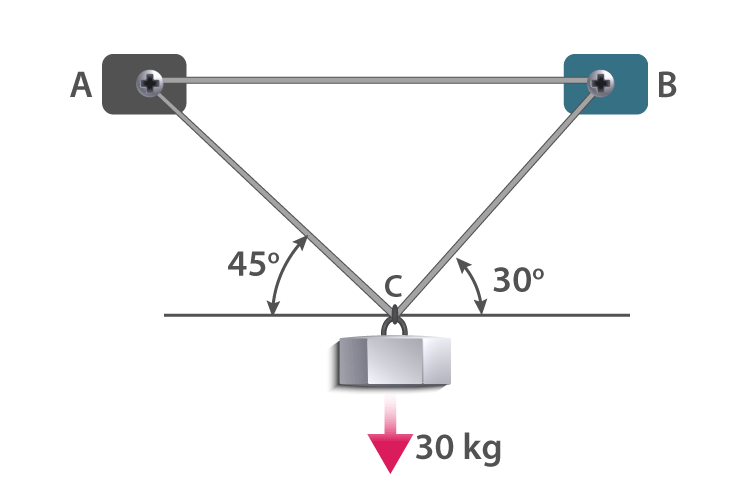Solution:

Given,

m = 30 kg, W = mg = 30 x 9.8 = 294 N

Let’s draw an FBD i.e. Free Body Diagram for the given condition. C be the point of suspension from where the iron block is hanging.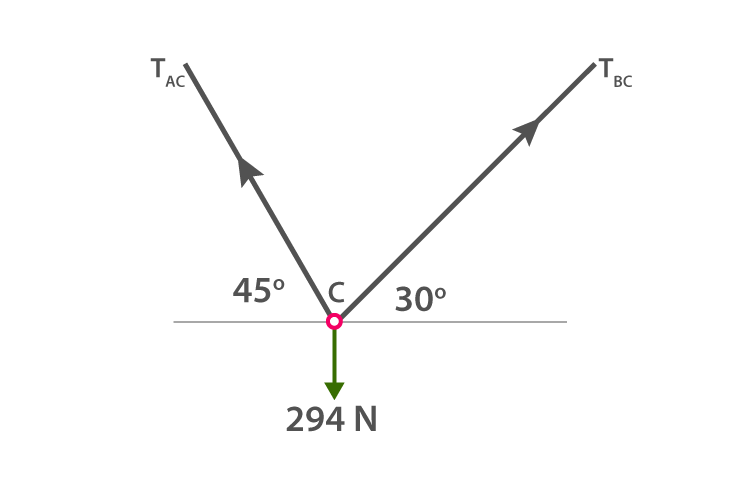We get required angles to apply Lami’s Theorem as,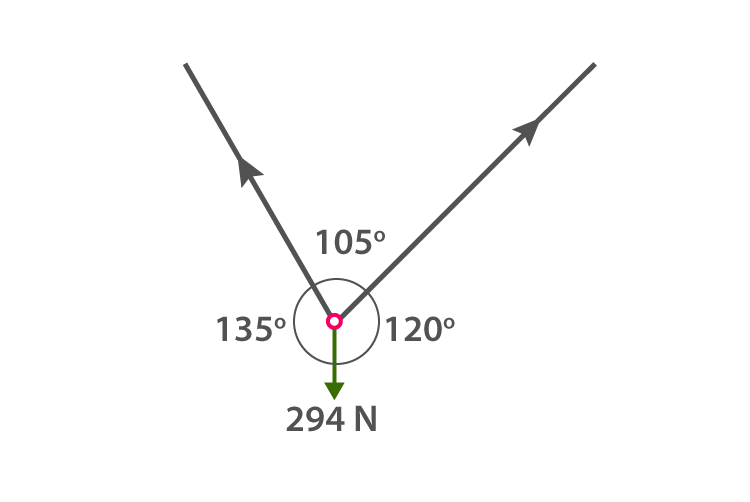Using Lami’s Formula we get,

$\frac{T_{AC}}{sin(120^{o})} = \frac{T_{BC}}{sin(135^{o})} = \frac{294}{sin(105^{o})}$

Therefore,

$\frac{T_{AC}}{sin(120^{o})} = \frac{294}{sin(105^{o})}$

i.e. $T_{AC} = \frac{294 \, X\, sin(120^{o})}{sin(105^{o})} = \frac{294 \, X\, 0.866}{0.966} = 263.566N$

Similarly,

$\frac{T_{BC}}{sin(120^{o})} = \frac{294}{sin(105^{o})}$

i.e. $T_{BC} = \frac{294 \, X\, sin(135^{o})}{sin(105^{o})} = \frac{294 \, X\, 0.707}{0.966} = 215.2N$

So, the required tensions along the shown directions are 263.566 N and 215.2 N respectively.

Example 4: Choose the correct alternative from the following.

A) Lami’s theorem is applicable to only regular-shaped bodies in equilibrium.

B) The theorem is derived on the basis of the cosine rule of trigonometry.

C) The theorem is very helpful in determining the unknown forces acting at a point for an object in equilibrium.

D) The theorem is useful in determining the forces acting on a moving object.

Solution: Option A mentions regular-shaped bodies in equilibrium, which is not true. In case the mass of irregularly shaped objects is given, the missing values of tension forces can be calculated. Option B mentions about cosine rule, which is not a correct statement. The theorem is derived on the basis of sine rule in a triangle which relates angles and opposite sides to those angles in a given triangle. Since the theorem is about the static equilibrium of objects, we do not apply it to moving objects even if they have a constant velocity. So, choice D is also incorrect.

We applied/used Lami’s Theorem to different situations in which objects are in equilibrium. So, option C gives us clear cut info when and where Lami’s Theorem is applied to find unknown forces.

Example 5: Which of the following is correct in the case of Lami’s Theorem?

A) When two coplanar forces acting at a point which is in equilibrium, then each force is proportional to the sine or the angle between the other two forces.

B) The theorem is applicable to randomly shaped 3D objects only.

C) There are three coplanar forces which act at a point which is in equilibrium, and each force is proportional to the sine or the angle between the other two forces.

D) Lami’s Theorem is helpful in determining a magnitude and direction of force acting on a moving object in a straight line.

Solution: First of all, Lami’s Theorem is applicable only in the case of coplanar concurrent forces. Secondly, it is applicable to planes as well as 3D objects. It means option B fails to satisfy the condition. There should be three concurrent forces acting at a point, so option A is invalid. Option D mentions force acting on a moving object in a straight line, which is not a correct situation to apply this theorem. So, option C which mentions the required conditions for the application of Lami’s Theorem is the right choice.